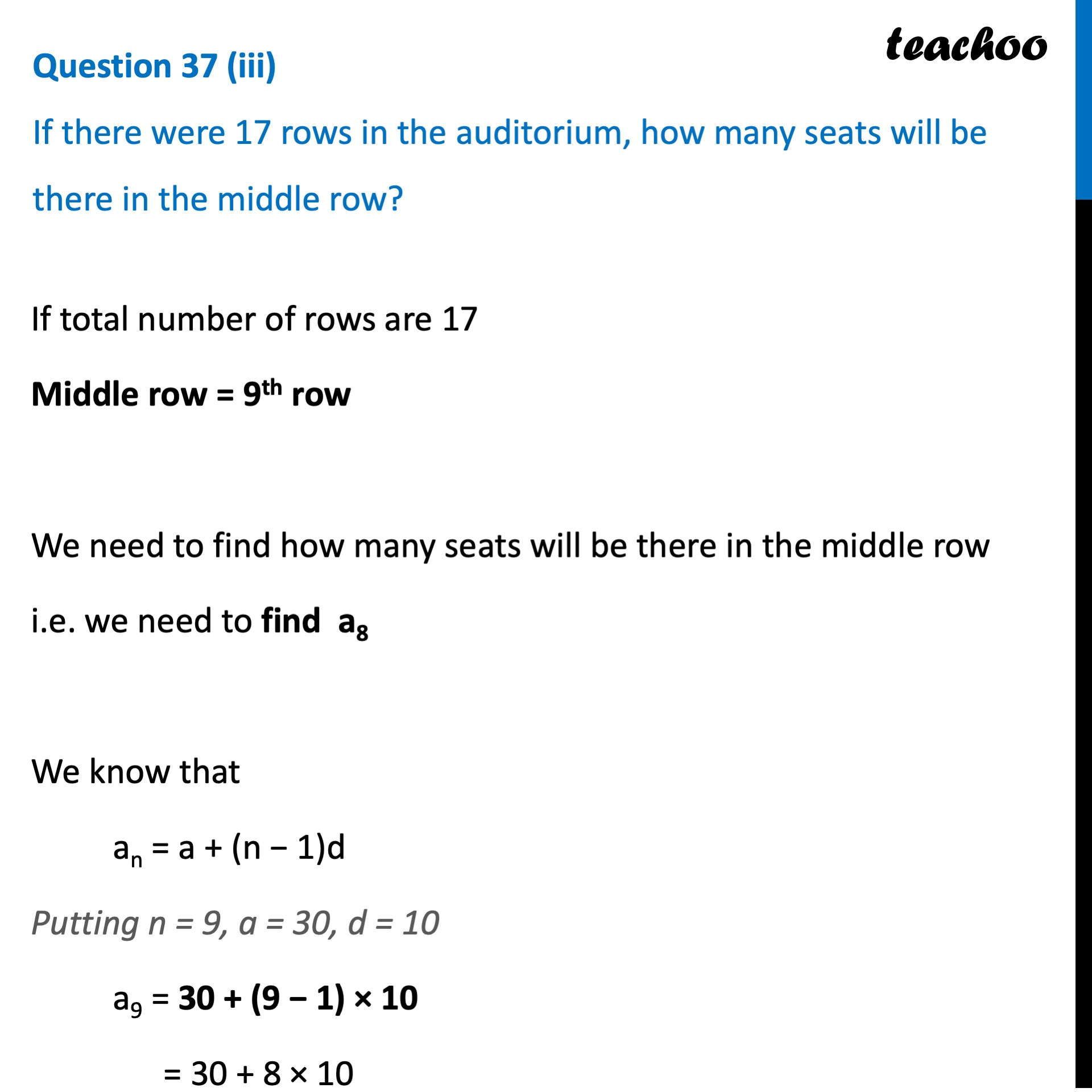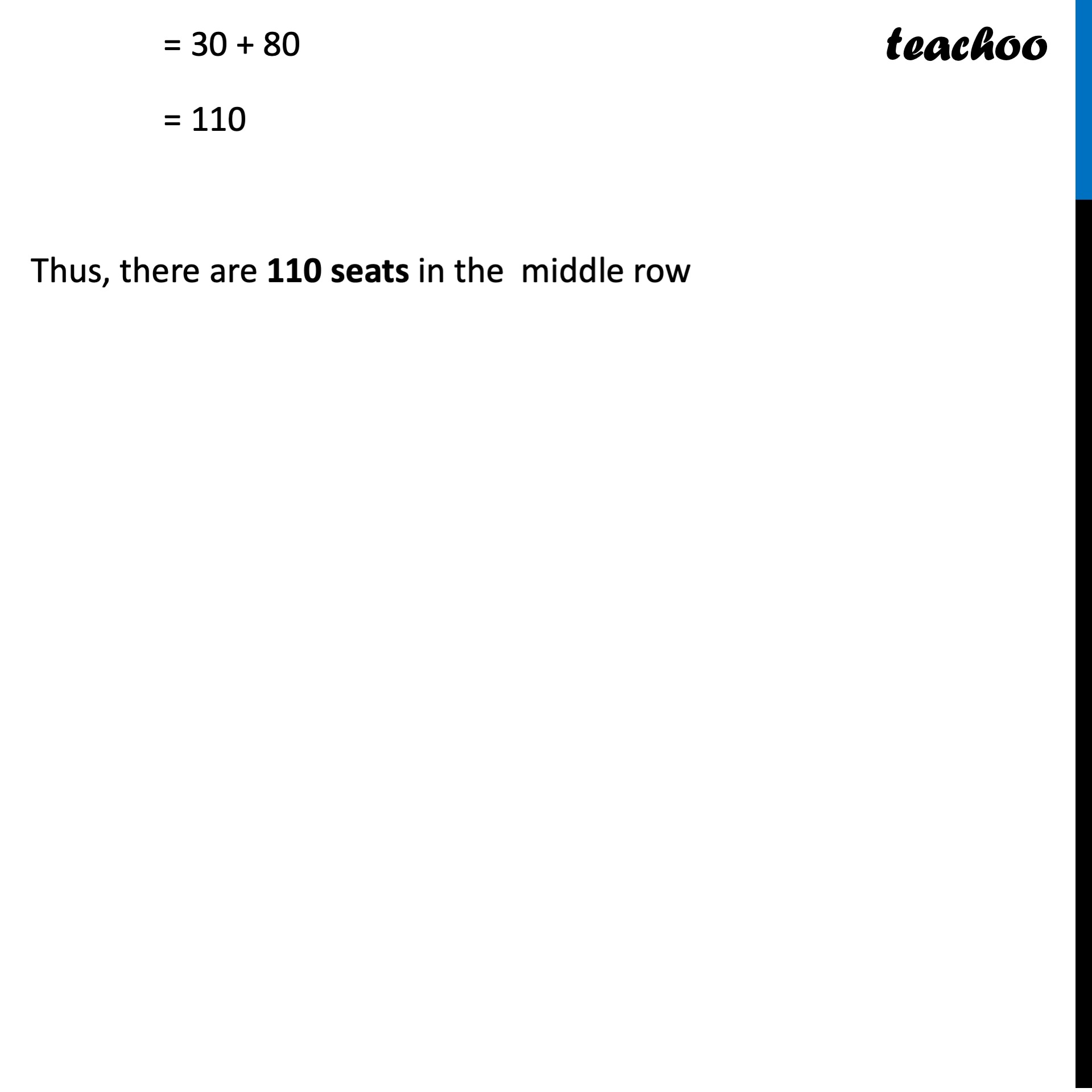CBSE Class 10 Sample Paper for 2023 Boards - Maths Standard

Class 10
Solutions of Sample Papers for Class 10 Boards

## If there were 17 rows in the auditorium, how many seats will be there in the middle row?Learn in your speed, with individual attention - Teachoo Maths 1-on-1 Class

### Transcript

Question 37 (iii) If there were 17 rows in the auditorium, how many seats will be there in the middle row? If total number of rows are 17 Middle row = 9th row We need to find how many seats will be there in the middle row i.e. we need to find a9 We know that an = a + (n − 1)d Putting n = 9, a = 30, d = 10 a8 = 30 + (9 − 1) × 10 = 30 + 8 × 10 = 30 + 80 = 110 Thus, there are 110 seats in the middle row